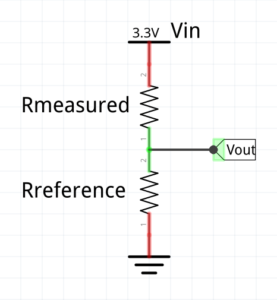# 3.9: Reading a Resistor Value

I used the ADC previously to read the internal sensors, so it’s simple to move on to external ones. Once you can read the value of a resistor, there is a wide choice of analog applications: thermistors, photocells, potentiometers, sliders, joysticks …

## Voltage Divider CircuitVout = Vin * Rref / (Rref + Rmeasured)

Assuming the ADC is configured for 12 bit precision and return a value in the range [0 … 4095]

Vin = VDDA

~VDDA~ * ADCRAW / 4095 = ~VDDA~ * Rref / (Rref + Rmeasured)

Rmeasured = Rref * (4095 / ADCRAW – 1)

## Devil in the whatnots

• Avoiding division by zero.

• Integer versus floating point calculation.

• Choosing the reference resistor.

• Calibration of the reference resistor.

``````#include <limits.h>
int R = ADCraw ? Rref * (4095 / ADCraw - 1) : INT_MAX ;
``````

`int R = ADCraw ? Rref * 4095 / ADCraw - Rref : INT_MAX ;`